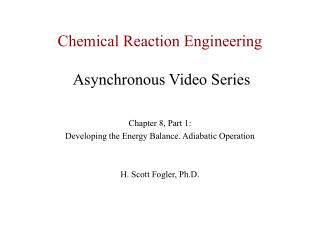Download PresentationChemical Reaction Engineering Asynchronous Video Series

# Chemical Reaction Engineering Asynchronous Video Series - PowerPoint PPT PresentationDownload Presentation## Chemical Reaction Engineering Asynchronous Video Series

- - - - - - - - - - - - - - - - - - - - - - - - - - - E N D - - - - - - - - - - - - - - - - - - - - - - - - - - -
##### Presentation Transcript

1. Chemical Reaction EngineeringAsynchronous Video Series Chapter 8, Part 1: Developing the Energy Balance. Adiabatic Operation H. Scott Fogler, Ph.D.

2. Energy Balances

3. Energy Balances

4. User Friendly Equations These equations relate X and T, and Fi and T

5. User Friendly Equations These equations relate X and T, and Fi and T

6. User Friendly Equations These equations relate X and T, and Fi and T

7. User Friendly Equations

8. User Friendly Equations

9. User Friendly Equations

10. User Friendly Equations

11. User Friendly Equations

12. Energy Balance

13. Energy Balance

14. Energy Balance

15. Energy Balance

16. Energy Balance

17. Energy Balance

18. Energy Balance

19. Energy Balance

20. Energy Balance

21. From Chapter 1 we have:

22. From Chapter 1 we have:

23. From Chapter 1 we have:

24. From Chapter 1 we have:

25. From Chapter 1 we have: Heat of Reaction

26. From Chapter 1 we have: Heat of Reaction

27. If Ti0=T0

28. Writing the energy balance in terms of conversion:

29. Writing the energy balance in terms of conversion:

30. Writing the energy balance in terms of conversion:

31. Writing the energy balance in terms of conversion:

32. Writing the energy balance in terms of conversion: Neglecting and rearranging:

33. Slope ≈ T X